CBSE Class 12 Sample Paper for 2020 Boards

Class 12
Solutions of Sample Papers and Past Year Papers - for Class 12 Boards

## A given quantity of metal is to be cast into a solid half circular cylinder with a rectangular base and semi-circular ends. Show that in order that total surface area is minimum, the ratio of length of cylinder to the diameter of semi-circular ends is π ∶ π + 2.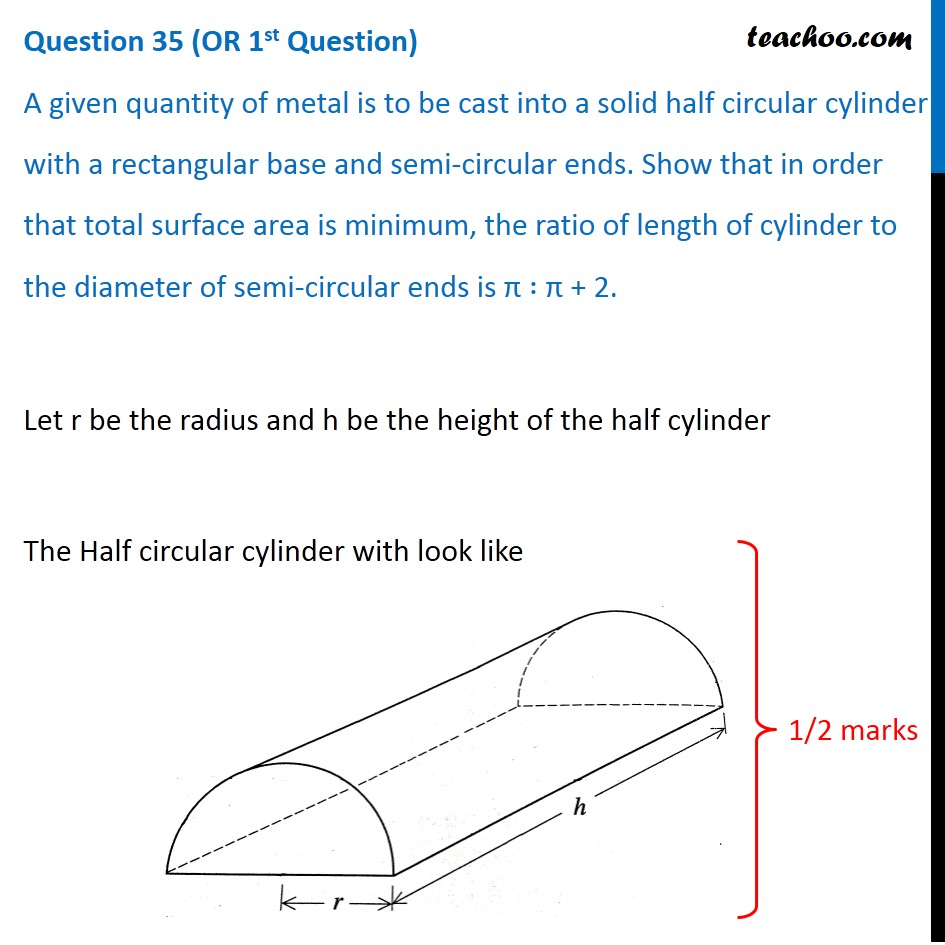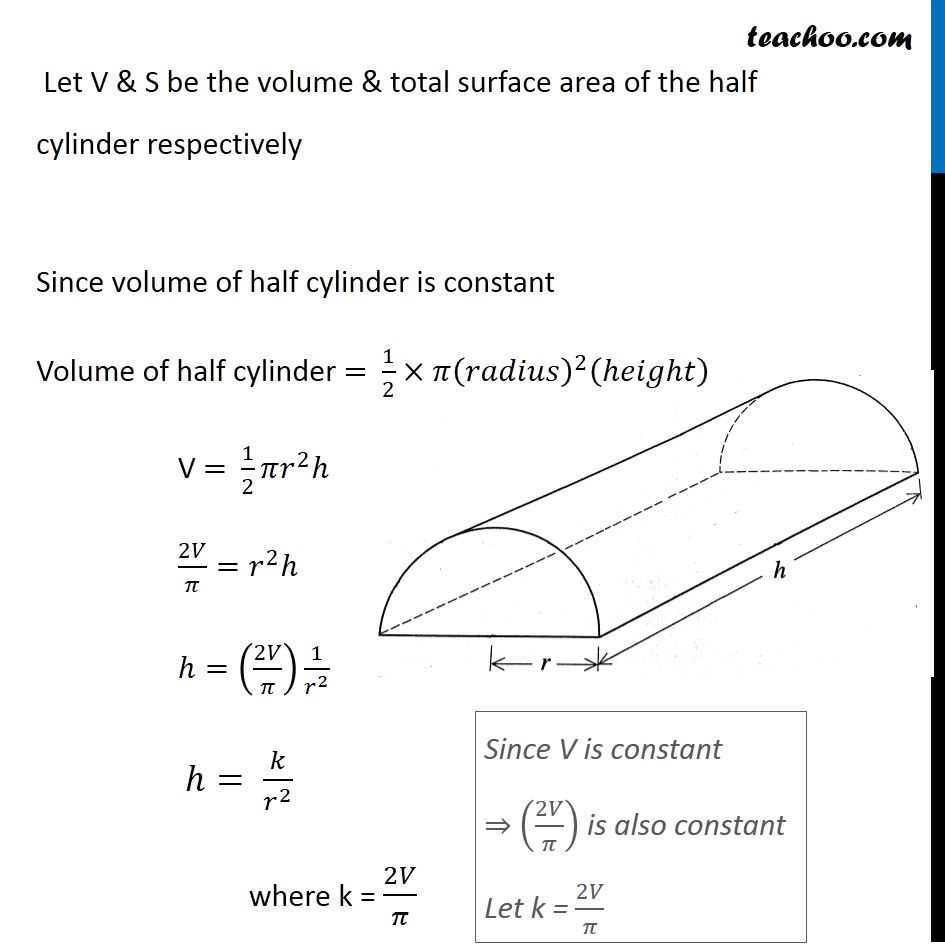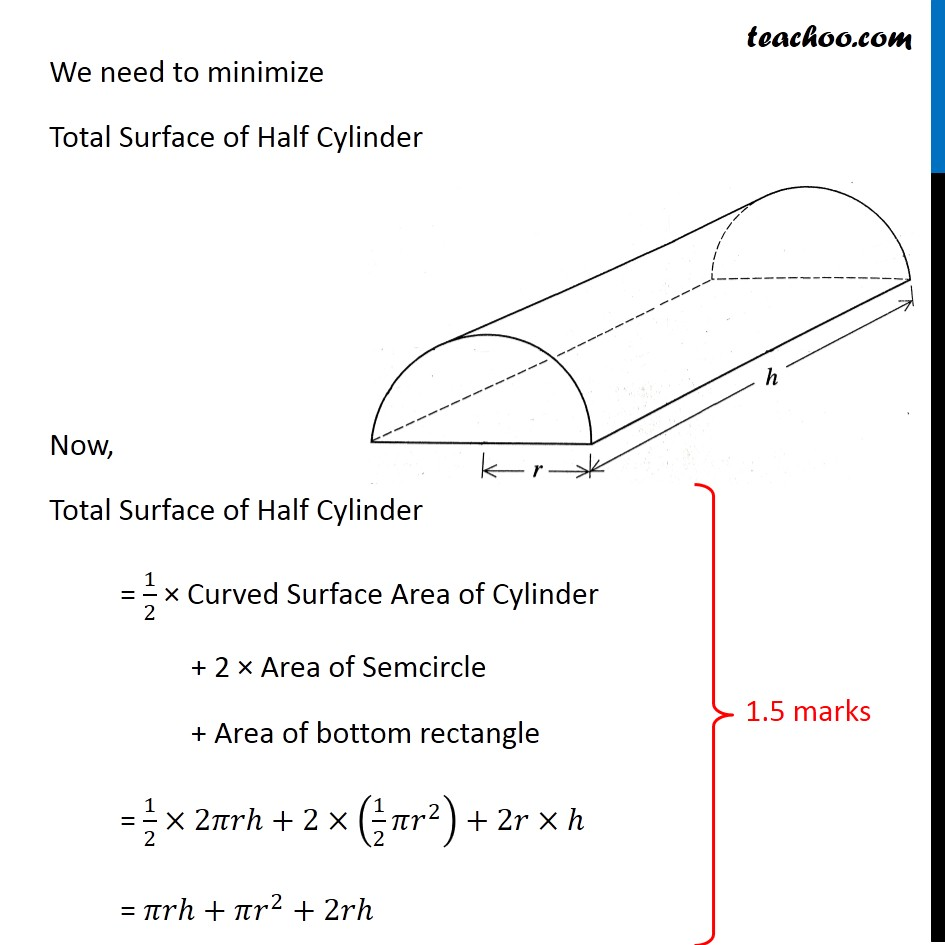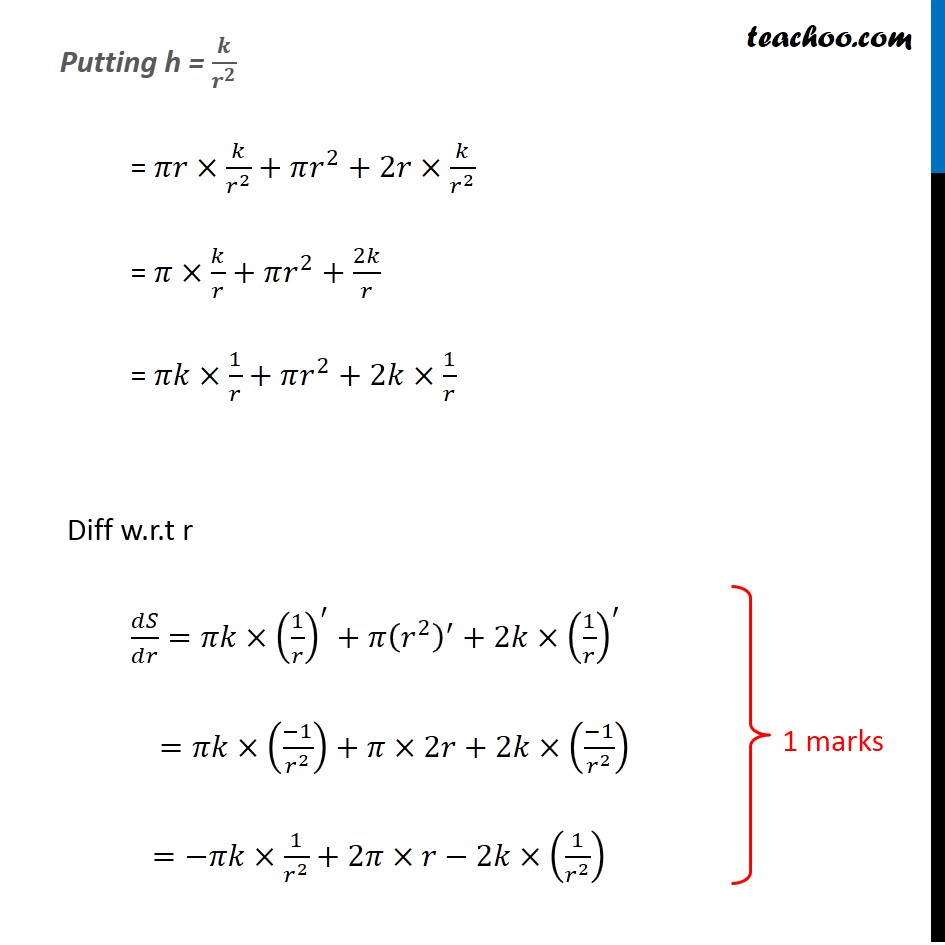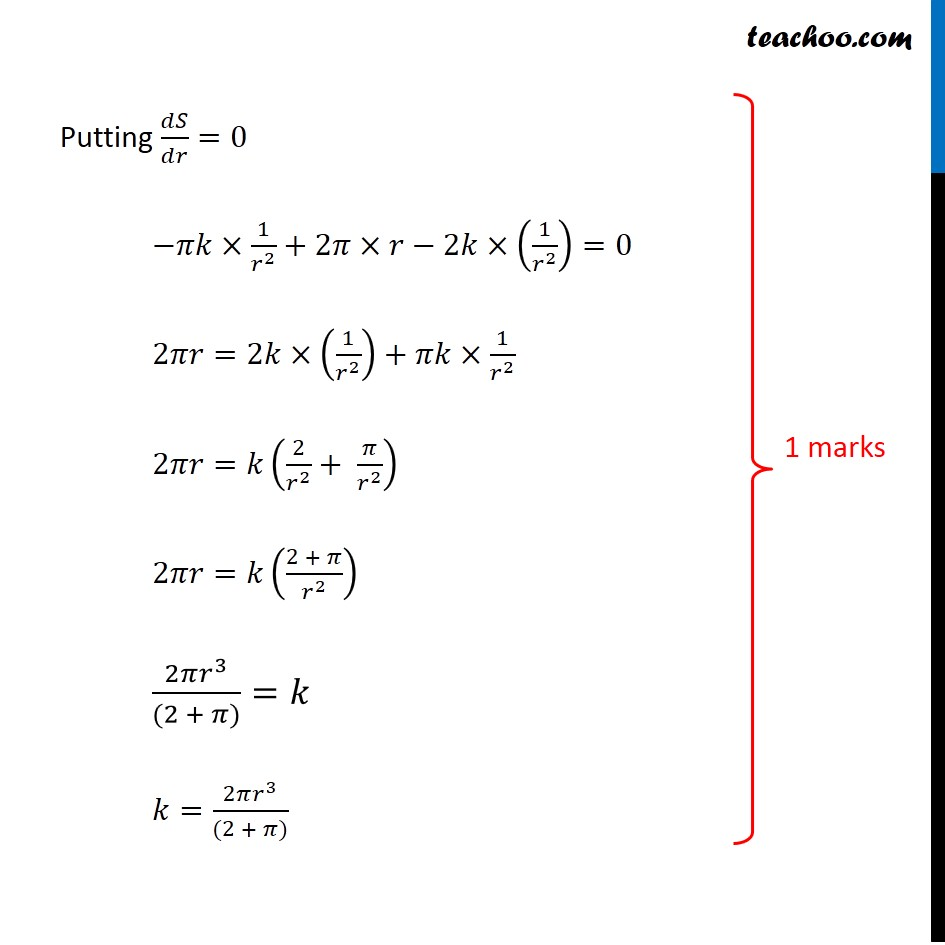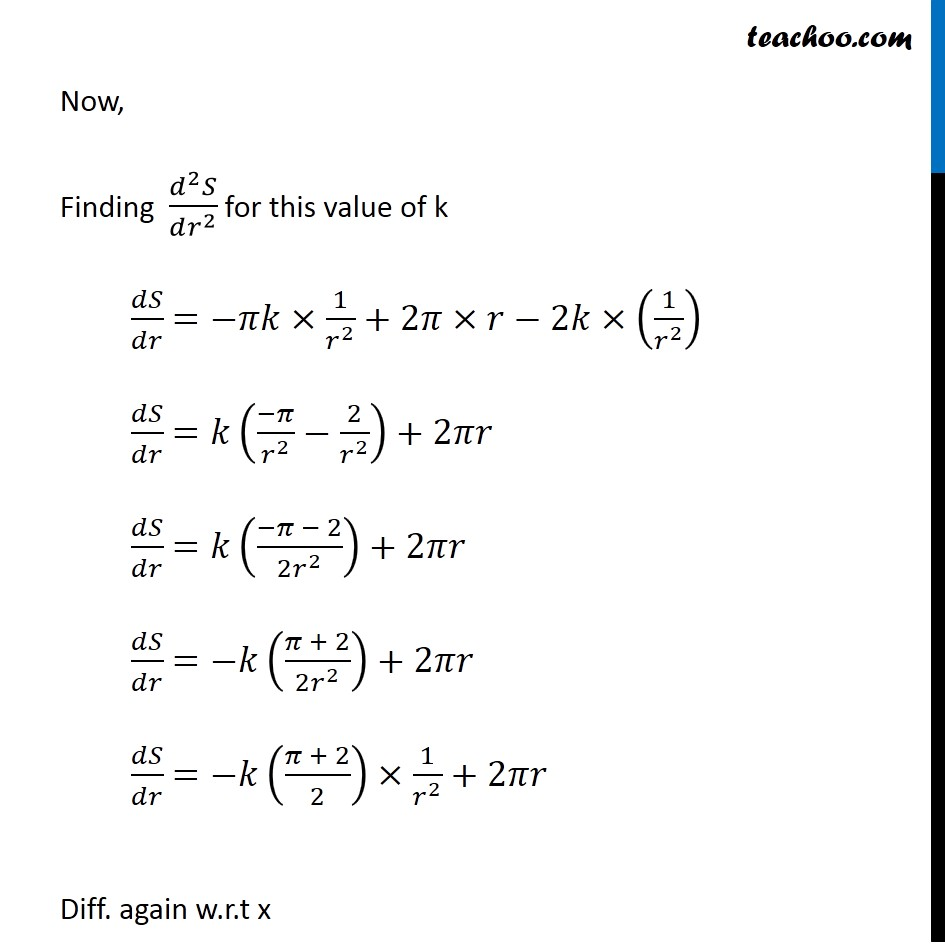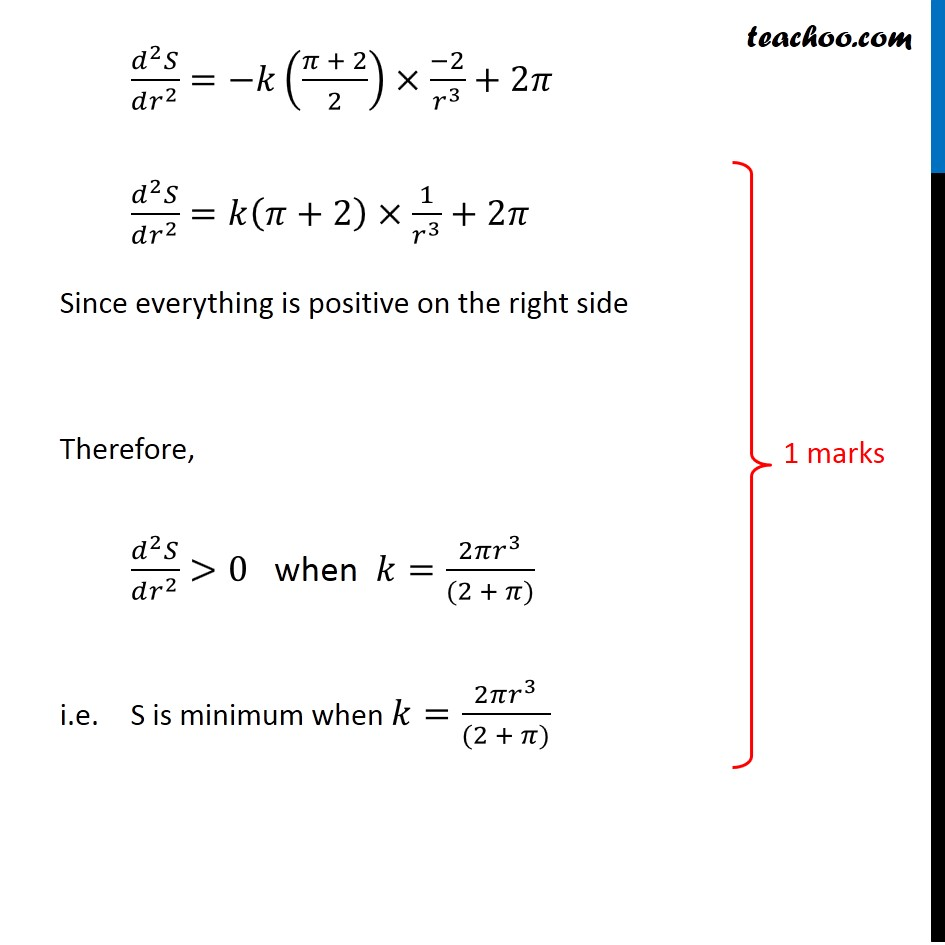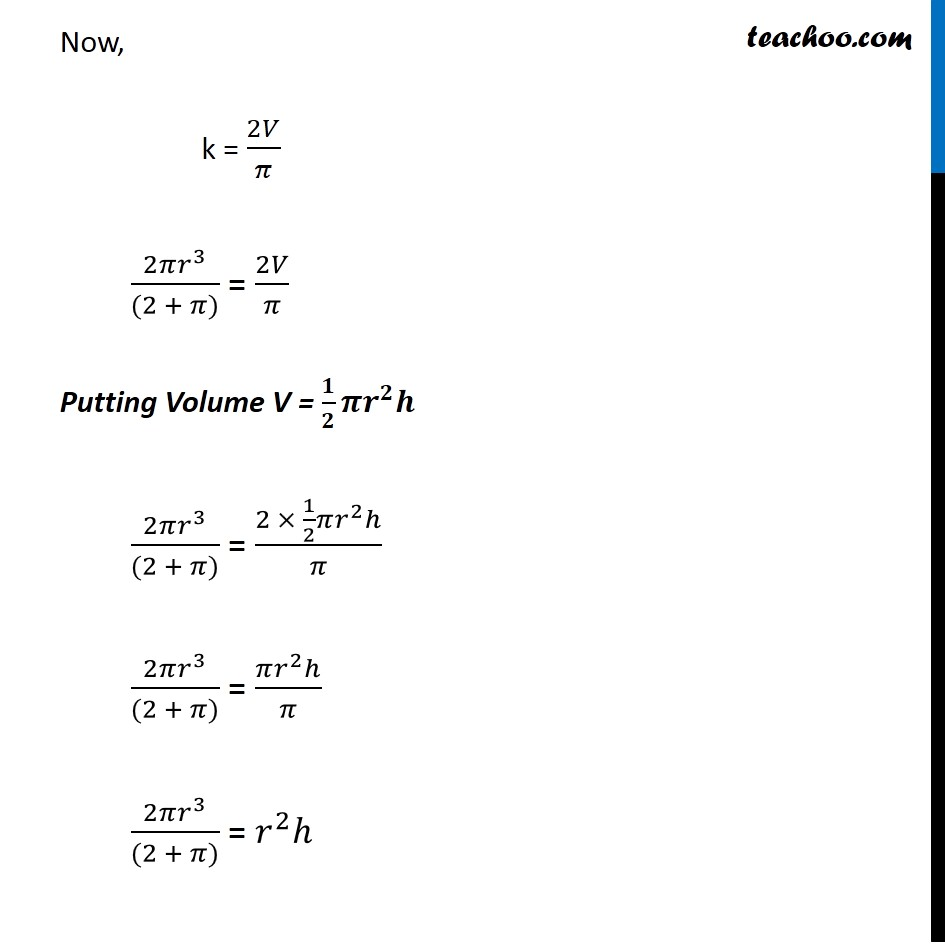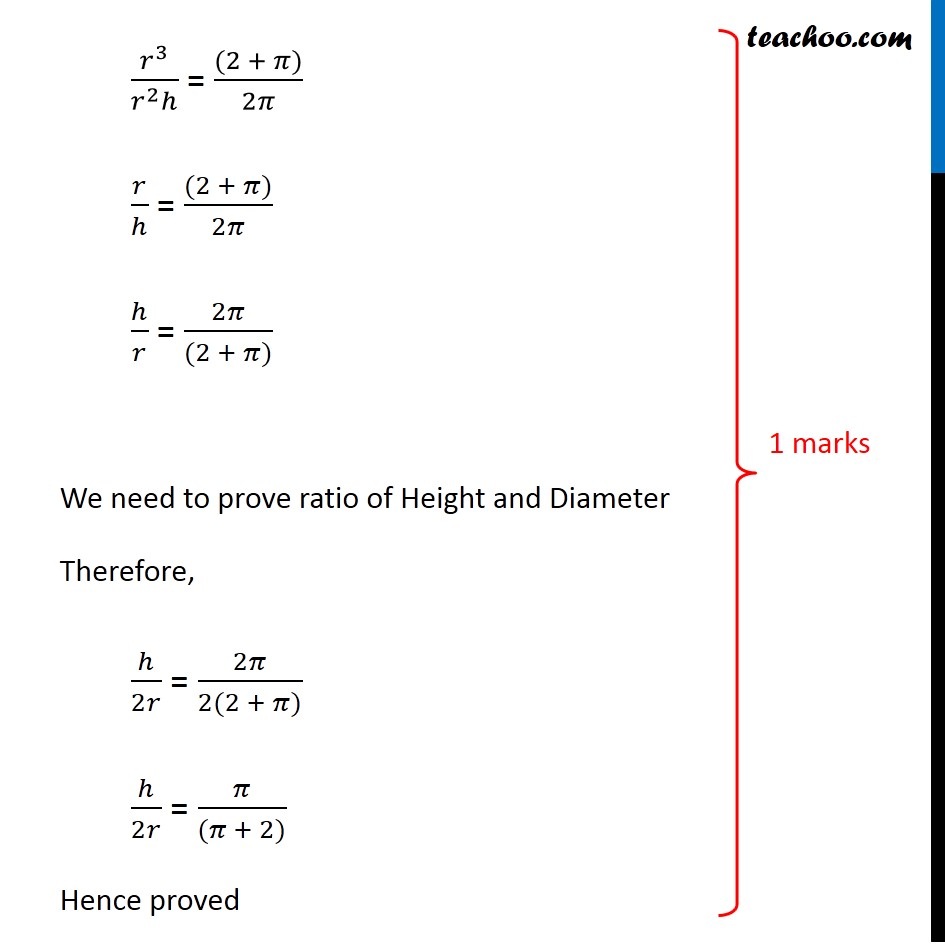Learn in your speed, with individual attention - Teachoo Maths 1-on-1 Class

### Transcript

Question 35 (OR 1st Question) A given quantity of metal is to be cast into a solid half circular cylinder with a rectangular base and semi-circular ends. Show that in order that total surface area is minimum, the ratio of length of cylinder to the diameter of semi-circular ends is π ∶ π + 2. Let r be the radius and h be the height of the half cylinder The Half circular cylinder with look like Let V & S be the volume & total surface area of the half cylinder respectively Since volume of half cylinder is constant Volume of half cylinder = 1/2×𝜋(𝑟𝑎𝑑𝑖𝑢𝑠)^2 (ℎ𝑒𝑖𝑔ℎ𝑡) V = 1/2 𝜋𝑟^2 ℎ 2𝑉/𝜋=𝑟^2 ℎ ℎ=(2𝑉/𝜋) 1/𝑟^2 ℎ= 𝑘/𝑟^2 where k = 2𝑉/𝜋 Since V is constant ⇒ (2𝑉/𝜋) is also constant Let k = 2𝑉/𝜋 We need to minimize Total Surface of Half Cylinder Now, Total Surface of Half Cylinder = 1/2 × Curved Surface Area of Cylinder + 2 × Area of Semcircle + Area of bottom rectangle = 1/2×2𝜋𝑟ℎ+2×(1/2 𝜋𝑟^2 )+2𝑟×ℎ = 𝜋𝑟ℎ+𝜋𝑟^2+2𝑟ℎ Putting h = 𝒌/𝒓^𝟐 = 𝜋𝑟×𝑘/𝑟^2 +𝜋𝑟^2+2𝑟×𝑘/𝑟^2 = 𝜋×𝑘/𝑟+𝜋𝑟^2+2𝑘/𝑟 = 𝜋𝑘×1/𝑟+𝜋𝑟^2+2𝑘×1/𝑟 Diff w.r.t r 𝑑𝑆/𝑑𝑟=𝜋𝑘×(1/𝑟)^′+𝜋(𝑟^2 )^′+2𝑘×(1/𝑟)^′ =𝜋𝑘×((−1)/𝑟^2 )+𝜋×2𝑟+2𝑘×((−1)/𝑟^2 ) =−𝜋𝑘×1/𝑟^2 +2𝜋×𝑟−2𝑘×(1/𝑟^2 ) Putting 𝑑𝑆/𝑑𝑟=0 −𝜋𝑘×1/𝑟^2 +2𝜋×𝑟−2𝑘×(1/𝑟^2 )=0 2𝜋𝑟=2𝑘×(1/𝑟^2 )+𝜋𝑘×1/𝑟^2 2𝜋𝑟=𝑘(2/𝑟^2 + 𝜋/𝑟^2 ) 2𝜋𝑟=𝑘((2 + 𝜋)/𝑟^2 ) (2𝜋𝑟^3)/((2 + 𝜋))=𝑘 𝑘=(2𝜋𝑟^3)/((2 + 𝜋)) Now, Finding (𝑑^2 𝑆)/(𝑑𝑟^2 ) for this value of k 𝑑𝑆/𝑑𝑟 =−𝜋𝑘×1/𝑟^2 +2𝜋×𝑟−2𝑘×(1/𝑟^2 ) 𝑑𝑆/𝑑𝑟 =𝑘((−𝜋)/𝑟^2 −2/𝑟^2 )+2𝜋𝑟 𝑑𝑆/𝑑𝑟 =𝑘((−𝜋 − 2)/(2𝑟^2 ))+2𝜋𝑟 𝑑𝑆/𝑑𝑟 =−𝑘((𝜋 + 2)/(2𝑟^2 ))+2𝜋𝑟 𝑑𝑆/𝑑𝑟 =−𝑘((𝜋 + 2)/2)×1/𝑟^2 +2𝜋𝑟 Diff. again w.r.t x (𝑑^2 𝑆)/(𝑑𝑟^2 )=−𝑘((𝜋 + 2)/2)×(−2)/𝑟^3 +2𝜋 (𝑑^2 𝑆)/(𝑑𝑟^2 )=𝑘(𝜋+2)×1/𝑟^3 +2𝜋 Since everything is positive on the right side Therefore, (𝑑^2 𝑆)/(𝑑𝑟^2 )>0 when 𝑘=(2𝜋𝑟^3)/((2 + 𝜋)) i.e. S is minimum when 𝑘=(2𝜋𝑟^3)/((2 + 𝜋)) Now, k = 2𝑉/𝜋 (2𝜋𝑟^3)/((2 + 𝜋)) = 2𝑉/𝜋 Putting Volume V = 𝟏/𝟐 𝝅𝒓^𝟐 𝒉 (2𝜋𝑟^3)/((2 + 𝜋)) = (2 × 1/2 𝜋𝑟^2 ℎ)/𝜋 (2𝜋𝑟^3)/((2 + 𝜋)) = (𝜋𝑟^2 ℎ)/𝜋 (2𝜋𝑟^3)/((2 + 𝜋)) = 𝑟^2 ℎ 𝑟^3/(𝑟^2 ℎ) = ((2 + 𝜋))/2𝜋 𝑟/ℎ = ((2 + 𝜋))/2𝜋 ℎ/𝑟 = 2𝜋/((2 + 𝜋)) We need to prove ratio of Height and Diameter Therefore, ℎ/2𝑟 = 2𝜋/(2(2 + 𝜋)) ℎ/2𝑟 = 𝜋/((𝜋 + 2)) Hence proved